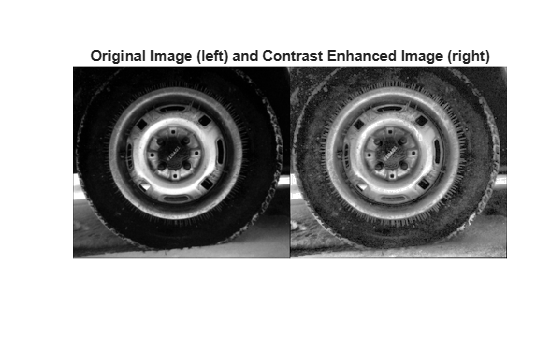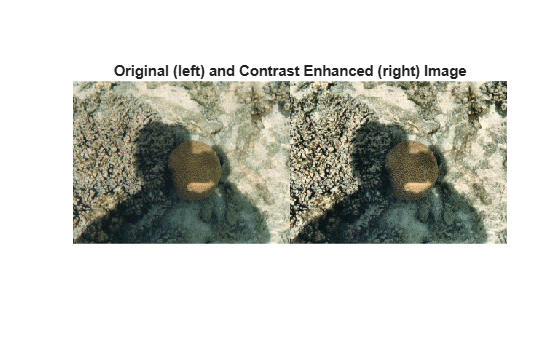## Syntax

``J = adapthisteq(I)``
``J = adapthisteq(I,Name,Value)``

## Description

example

````J = adapthisteq(I)` enhances the contrast of the grayscale image `I` by transforming the values using contrast-limited adaptive histogram equalization (CLAHE) .```
````J = adapthisteq(I,Name,Value)` uses name-value pairs to control aspects of the contrast enhancement.```

## Examples

collapse all

Apply CLAHE to an image and display the results.

```I = imread('tire.tif'); J = adapthisteq(I,'clipLimit',0.02,'Distribution','rayleigh'); imshowpair(I,J,'montage'); title('Original Image (left) and Contrast Enhanced Image (right)')```Read the indexed color image into the workspace.

`[X, MAP] = imread('shadow.tif');`

Convert the indexed image into a truecolor (RGB) image, then convert the RGB image into the L*a*b* color space.

```RGB = ind2rgb(X,MAP); LAB = rgb2lab(RGB);```

Scale values to the range expected by the `adapthisteq` function, [0 1].

`L = LAB(:,:,1)/100;`

Perform CLAHE on the L channel. Scale the result to get back to the range used by the L*a*b* color space.

```L = adapthisteq(L,'NumTiles',[8 8],'ClipLimit',0.005); LAB(:,:,1) = L*100;```

Convert the resulting image back into the RGB color space.

`J = lab2rgb(LAB);`

Display the original image and the processed image.

```figure imshowpair(RGB,J,'montage') title('Original (left) and Contrast Enhanced (right) Image')```Shadows in the enhanced image look darker and highlights look brighter. The overall contrast is improved.

## Input Arguments

collapse all

Grayscale image, specified as a 2-D numeric matrix.

Data Types: `single` | `double` | `int16` | `uint8` | `uint16`

### Name-Value Pair Arguments

Specify optional comma-separated pairs of `Name,Value` arguments. `Name` is the argument name and `Value` is the corresponding value. `Name` must appear inside quotes. You can specify several name and value pair arguments in any order as `Name1,Value1,...,NameN,ValueN`.

Example: `'NumTiles',[8 16]` divides the image into 8 rows and 16 columns of tiles.

Number of rectangular contextual regions (tiles) into which `adapthisteq` divides the image, specified as a 2-element vector of positive integers. With the original image divided into `M` rows and `N` columns of tiles, the value of `'NumTiles'` is `[M N]`. Both `M` and `N` must be at least `2`. The total number of tiles is equal to `M*N`. The optimal number of tiles depends on the type of the input image, and it is best determined through experimentation.

Data Types: `double`

Contrast enhancement limit, specified as a number in the range [0, 1]. Higher limits result in more contrast.

`'ClipLimit'` is a contrast factor that prevents oversaturation of the image specifically in homogeneous areas. These areas are characterized by a high peak in the histogram of the particular image tile due to many pixels falling inside the same gray level range. Without the clip limit, the adaptive histogram equalization technique could produce results that, in some cases, are worse than the original image.

Data Types: `double`

Number of histogram bins used to build a contrast enhancing transformation, specified as a positive integer. Higher values result in greater dynamic range at the cost of slower processing speed.

Data Types: `double`

Range of the output image data, specified as one of these values.

ValueDescription
`'full'`Use the full range of the output class (such as [0 255] for `uint8`).
`'original'`Limit the range to ```[min(I(:)) max(I(:))]```.

Data Types: `char` | `string`

Desired histogram shape, specified as one of the following values:

ValueDescription
`'uniform'`Create a flat histogram.
`'rayleigh'`Create a bell-shaped histogram.
`'exponential'`Create a curved histogram.

`'Distribution'` specifies the distribution that `adapthisteq` uses as the basis for creating the contrast transform function. The distribution you select should depend on the type of the input image. For example, underwater imagery appears to look more natural when the Rayleigh distribution is used.

Data Types: `char` | `string`

Distribution parameter, specified as a nonnegative number. `'Alpha'` is only used when `'Distribution'` is set to `'rayleigh'` or `'exponential'`.

Data Types: `double`

## Output Arguments

collapse all

Contrast enhanced image, returned as a 2-D matrix of the same data type as the input image `I`.

## Algorithms

CLAHE operates on small regions in the image, called tiles, rather than the entire image. `adapthisteq` calculates the contrast transform function for each tile individually. Each tile's contrast is enhanced, so that the histogram of the output region approximately matches the histogram specified by the `'``Distribution``'` value. The neighboring tiles are then combined using bilinear interpolation to eliminate artificially induced boundaries. The contrast, especially in homogeneous areas, can be limited to avoid amplifying any noise that might be present in the image.

 Zuiderveld, Karel. “Contrast Limited Adaptive Histograph Equalization.” Graphic Gems IV. San Diego: Academic Press Professional, 1994. 474–485.